## Applying the Criteria for Aromaticity

Valence bond theory uses Lewis diagrams to depict structure and bonding of covalent entities, such as molecules and polyatomic ions, henceforth molecules. The Lewis diagram of many a molecule, however, is not consistent with the observed properties of the molecule.  The Lewis diagram of some molecules suggests a ring bearing a fully conjugated $\pi$-electron system, or loop of $\pi$ electrons, provided each atom in the ring is sp2– or sp-hybridized.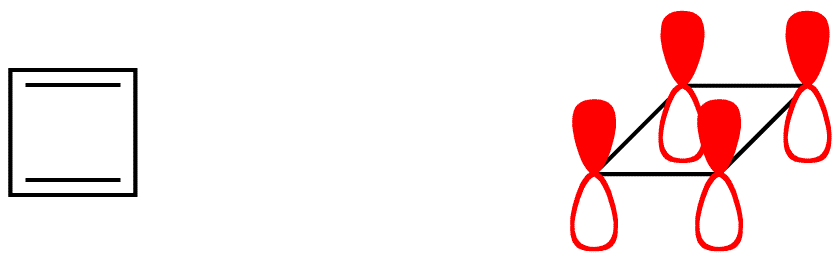• benzene (2)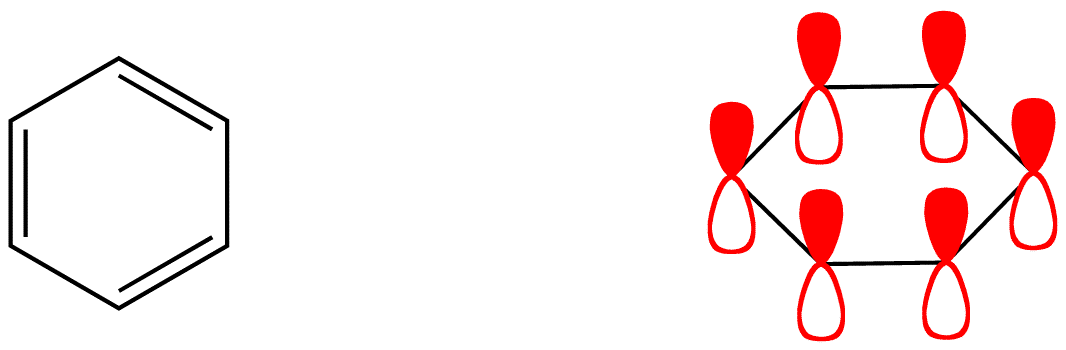• cyclooctatetraene (3)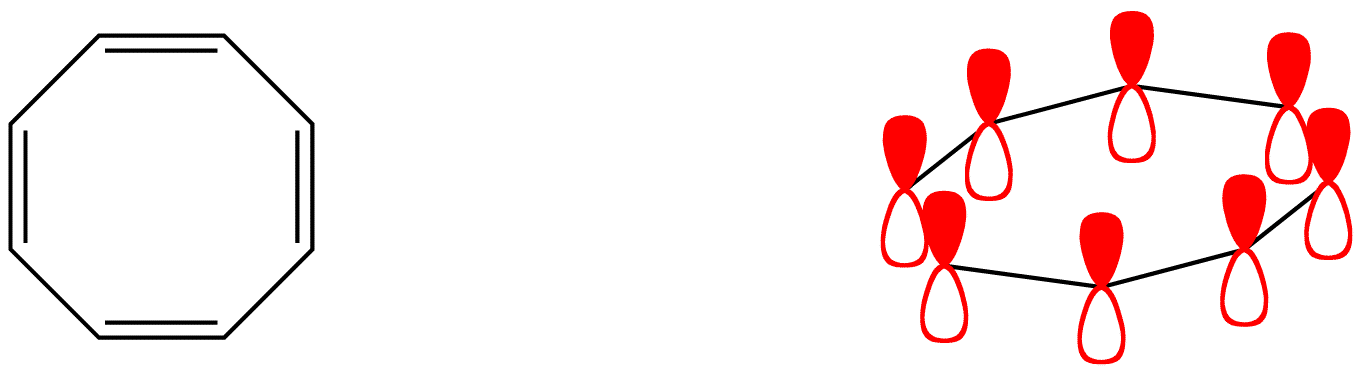• pyridine (4)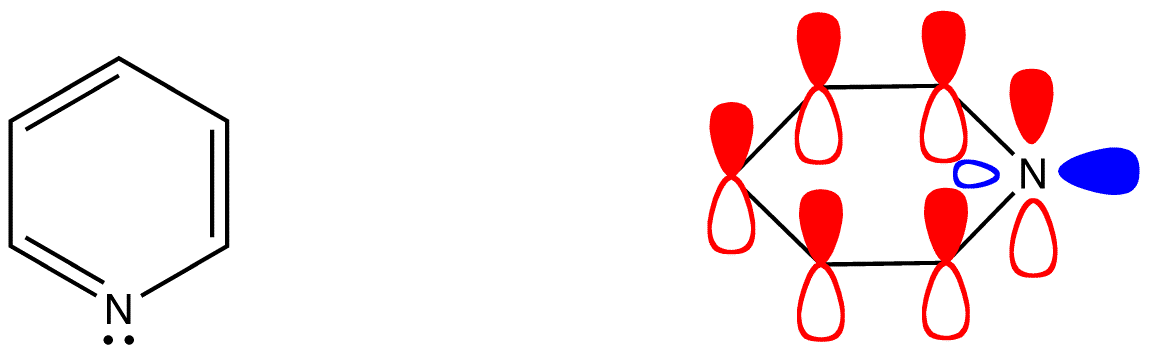• pyrrole (5)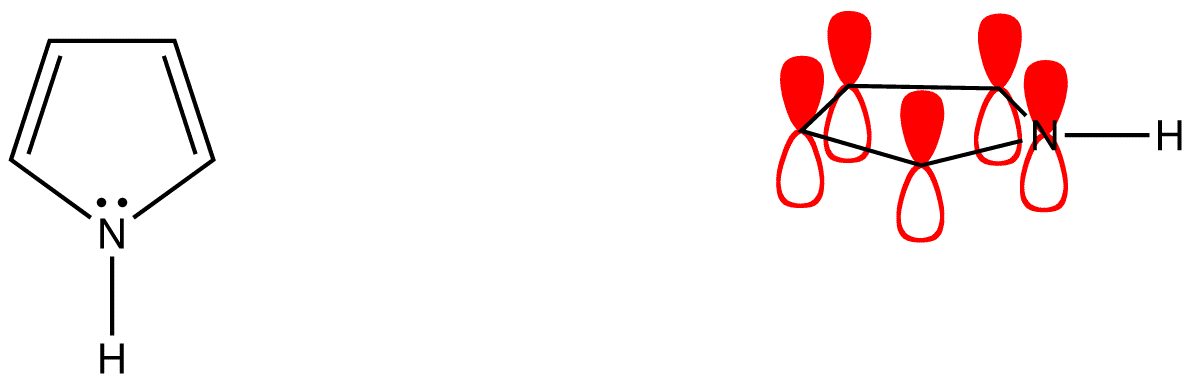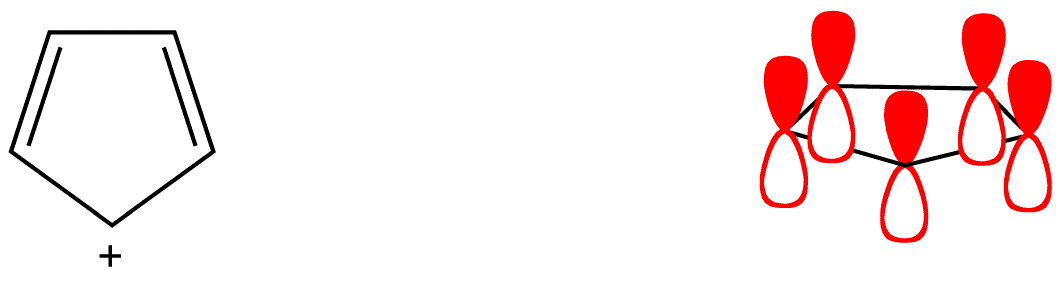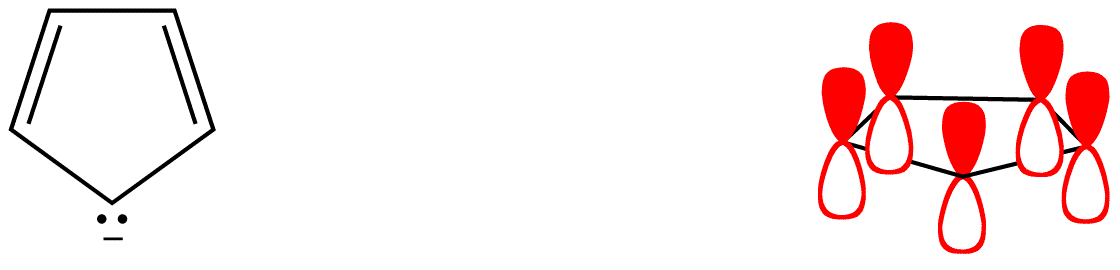As evident from the stability of $\pi$ electrons however, only some such rings actually have a loop of $\pi$ electrons. Of the above examples, only the rings in 2, 4, 5, and 7 have a loop of $\pi$ electrons. Resonance theory can predict that the rings in 2, 4, 5, and 7 have a loop of $\pi$ electrons.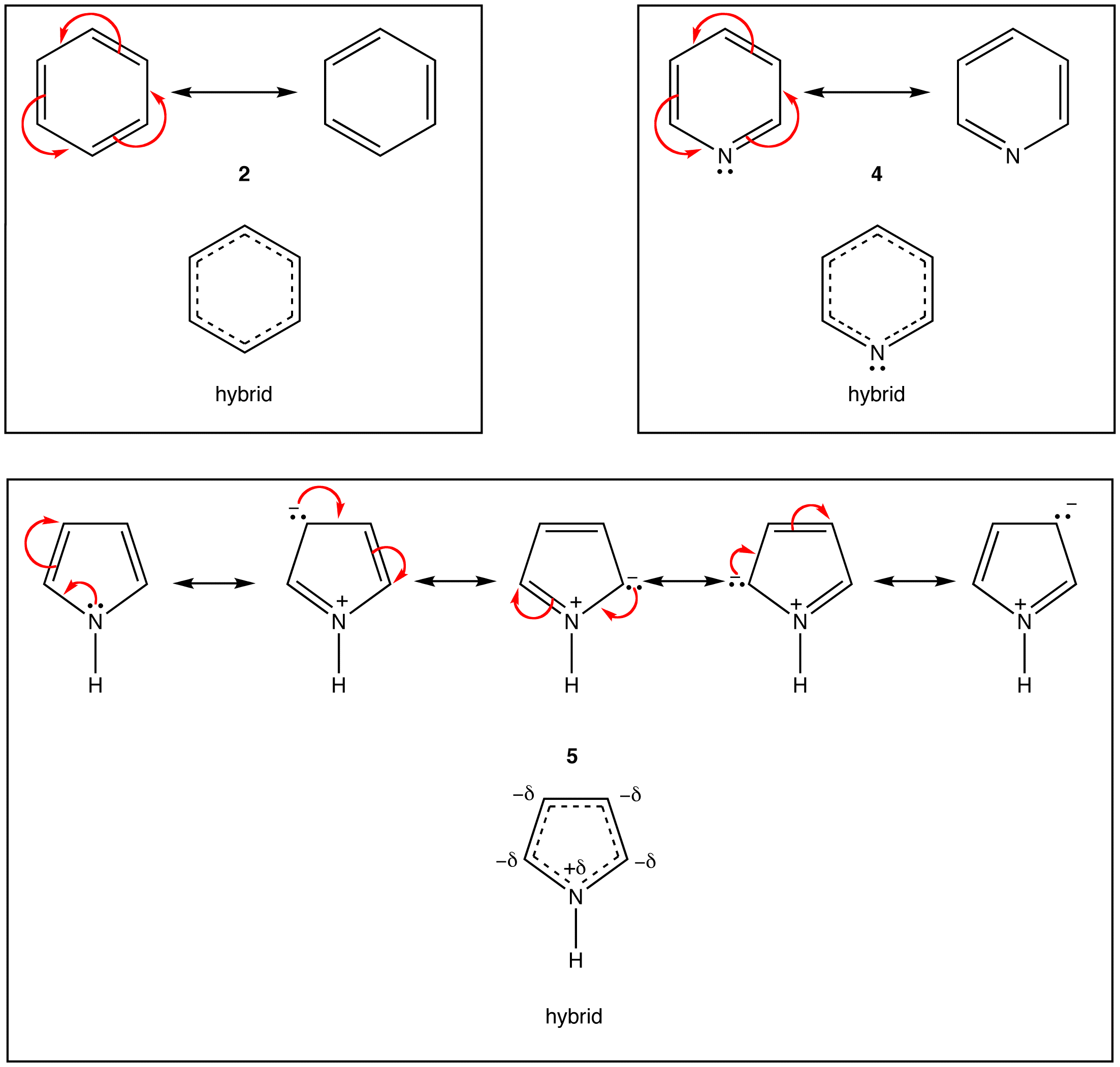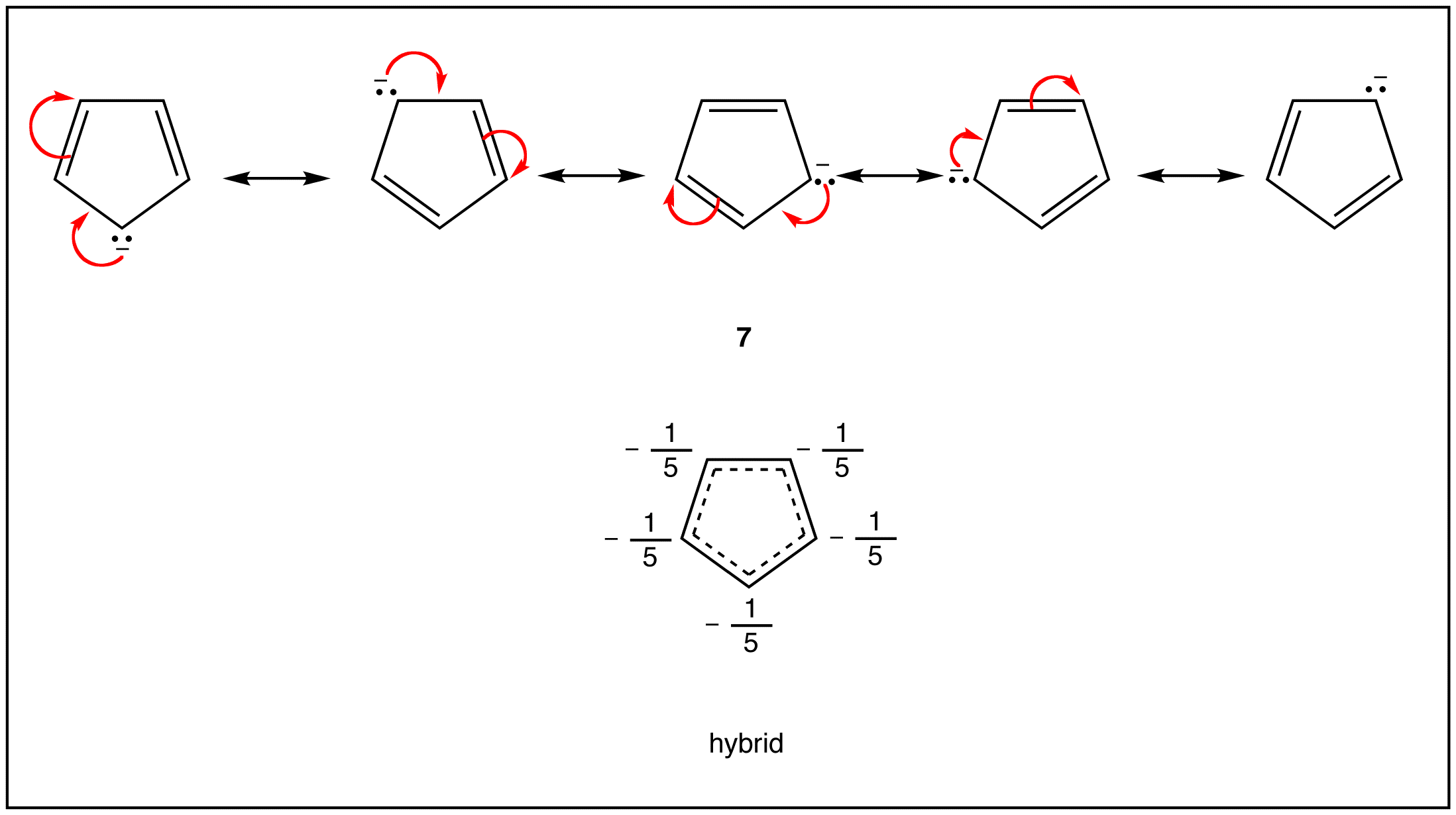According to resonance theory, however, the rings in 1, 3, and 6 also should have a loop of $\pi$ electrons.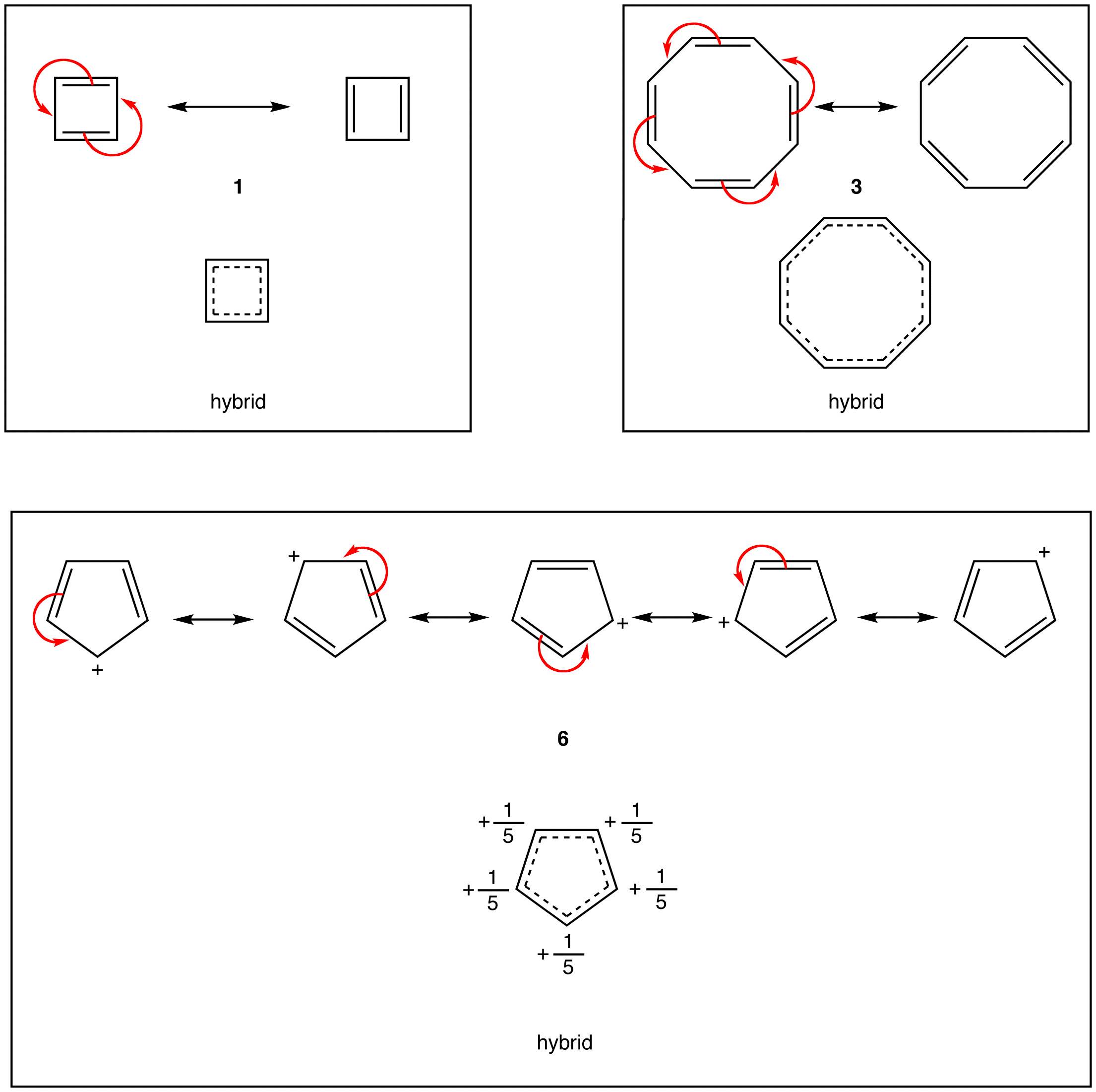Evidently, resonance theory is no more reliable a tool than Lewis diagrams to be used in predicting if a ring has a loop of $\pi$ electrons. Rings whose Lewis diagram implies a loop of $\pi$ electrons can be classified into three classes:

1. aromatic rings
2. antiaromatic rings
3. nonaromatic rings

To find whether a ring is aromatic, antiaromatic, nonaromatic, or none of the above, use the following flow chart.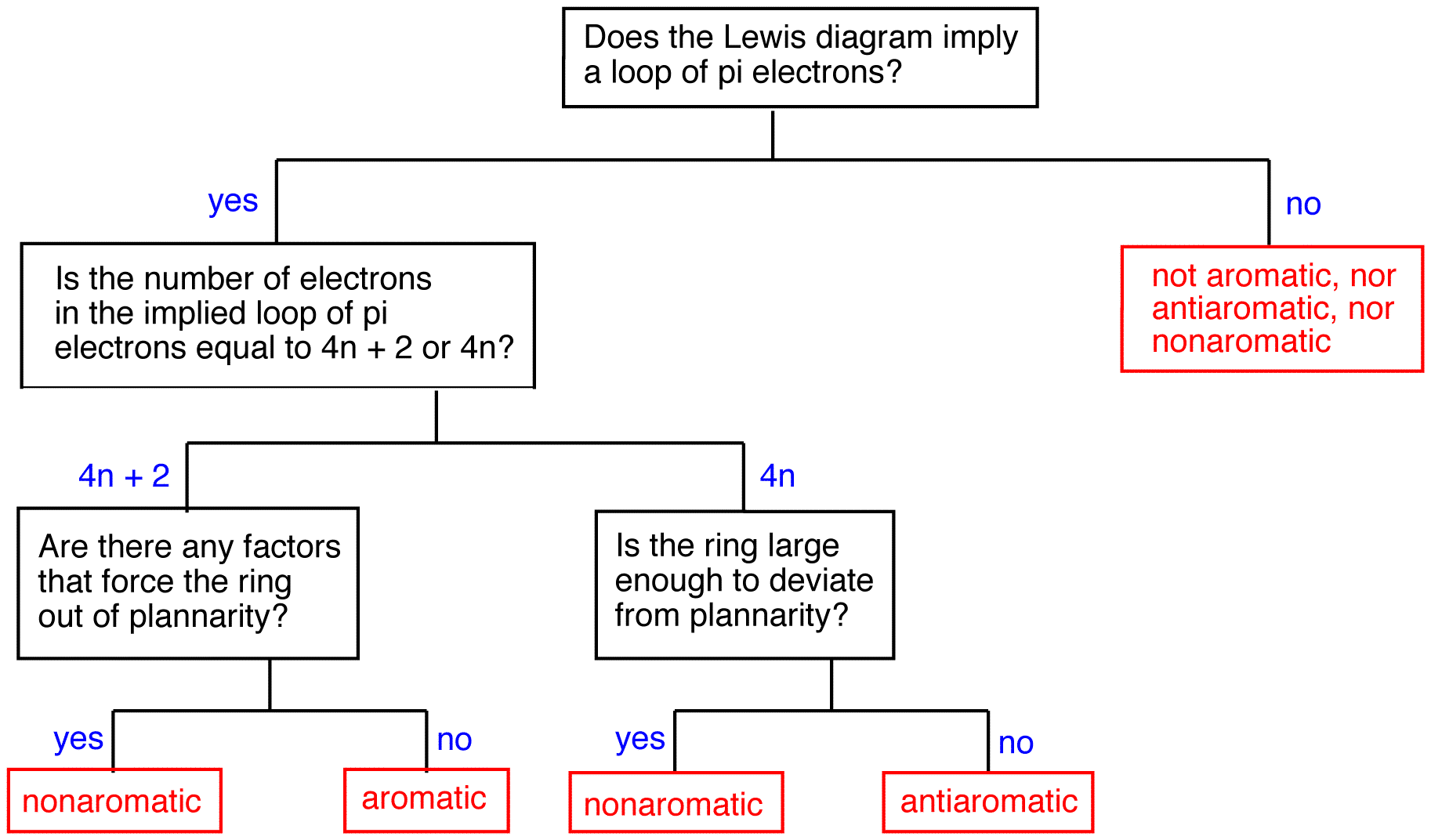Figure 1.: Flowchart for determining aromatic behavior in cyclic molecules.

### Aromatic rings

A ring that does have a loop of $\pi$ electrons is said to be aromatic. Thus, the rings in 2, 4, 5, and 7 are aromatic. A compound whose molecule contains one or more aromatic rings is called an aromatic compound. Thus, 2, 4, 5, and 7 are aromatic compounds. There are chemical and spectroscopic methods that can be used to experimentally determine whether a ring is aromatic or not. All aromatic rings share two structural features:

1.  The ring is planar.
2. The ring obeys the Hückel’s rule, which states that the number of electrons in the loop of $\pi$ electrons is equal to 4n + 2, where n is zero or a positive whole number.As is evident from the above examples, determining whether a ring obeys the Hückel’s rule or not using the Lewis diagram is straightforward.  However, determining whether a ring is planar or not requires careful consideration of many factors. A case in point is annulene (10).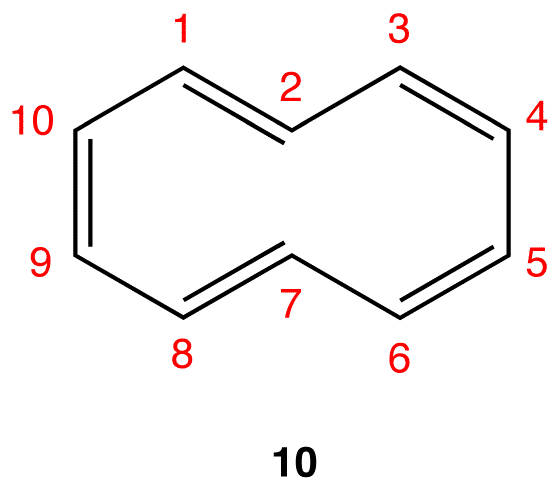If the ring in 10, which obeys the Hückel’s Rule, were planar, the hydrogen atoms on C-2 and C-7, which are inside the ring, would have to occupy the same space, which would be physically impossible.  To accommodate the hydrogen atoms on C-2 and C-7 inside the ring, the ring is forced to deviate from planarity. Thus, 10 is not aromatic. If annulene were to assume a configuration in which all double bonds were cis double bonds (11), the ring would be planar and, therefore, be aromatic.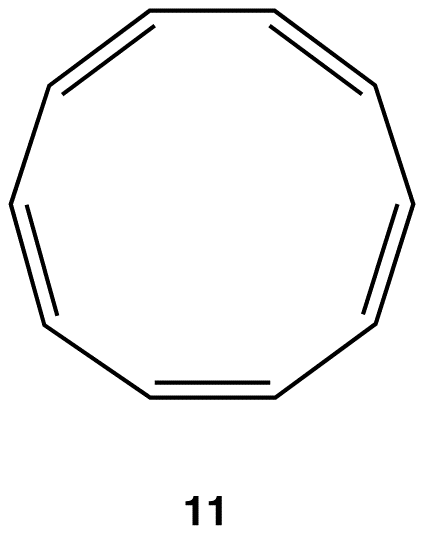Experimentally, however, annulene exists exclusively as 10, indicating that the stability gained by having a loop of $\pi$ electrons in 11 is outweighed by considerable angle strain.

### Antiaromatic rings

A ring that does not have a loop of $\pi$ electrons and is extremely unstable is said to be antiaromatic. A compound whose molecule contains one or more antiaromatic rings is called an antiaromatic compound.  The rings in 1 and 6 are antiaromatic. 1 can be isolated only in an inert, solid matrix (helium, argon, etc.) at temperatures approaching absolute zero.  Under all other conditions, it dimerizes rapidly.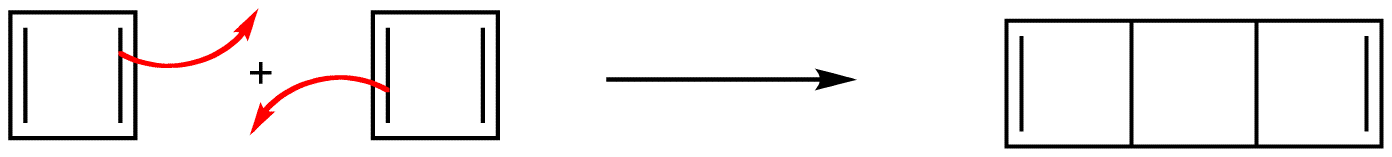Experiments have shown that the ring in 1 is not a square but a rectangle, which is evidence that the ring in 1 does not have a loop of $\pi$ electrons. According to molecular orbital theory, 1 is a diradical, which is consistent with its extreme reactivity. 6 has never been observed.

All antiaromatic rings share two features:

1. The ring is planar.
2. If the ring had a loop of $\pi$ electrons as implied by the Lewis diagram, the number of electrons in the loop would be equal to 4n, where n is zero or a positive whole number.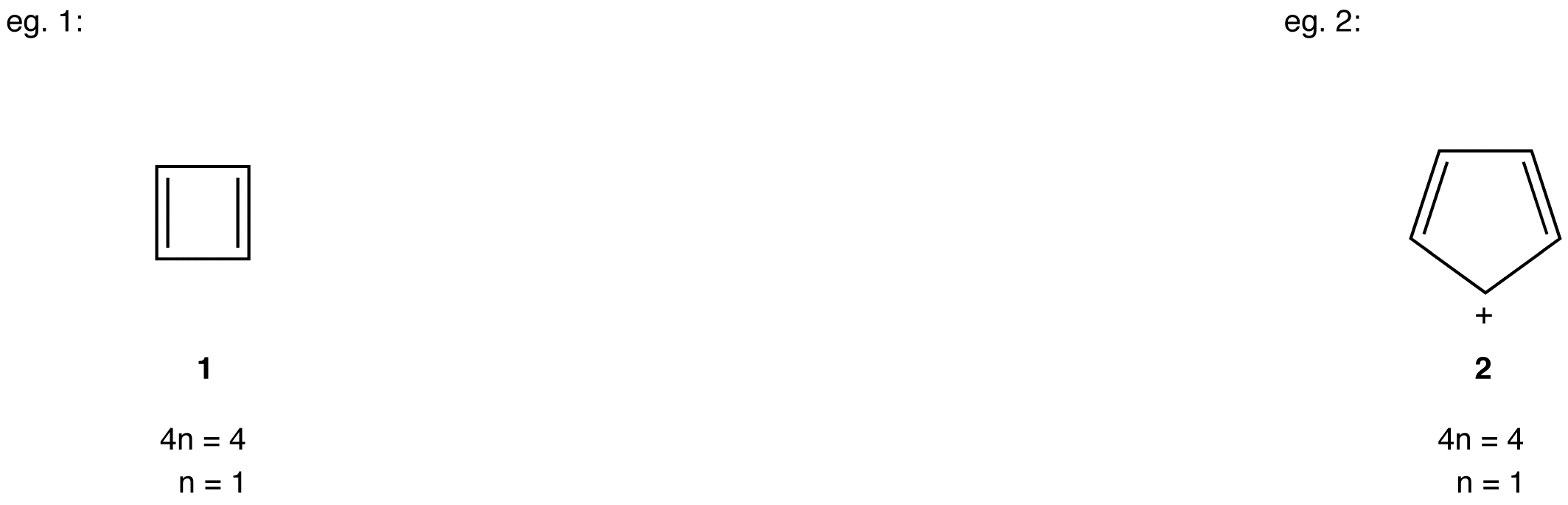Large and, therefore, flexible rings whose Lewis structure suggests a loop of $\pi$ electrons containing 4n number of electrons avoid being antiaromatic by deviating from planarity.

eg: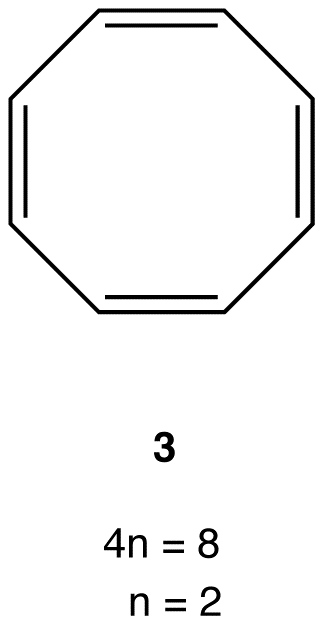Experimentally, 3 is about as stable as any typical alkene, meaning 3 is not antiaromatic. There are no antiaromatic rings containing more than five atoms.

### Nonaromatic rings

A ring that does not have a loop of $\pi$ electrons but is not antiaromatic is said to be nonaromatic. A compound whose molecule contains one of more nonaromatic rings is called a nonaromatic compound. A ring whose $\pi$ system contains a 4n number of electrons but avoids being antiaromatic by deviating from planarity is nonaromatic. For example,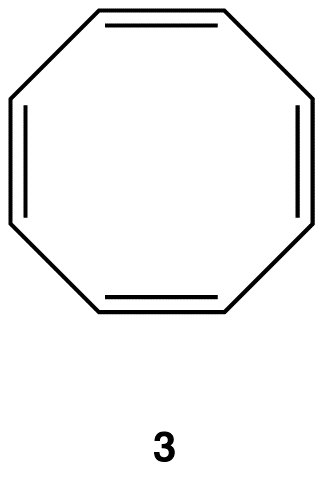Experimentally, 3 exists in the following nonplanar conformation, known as the tub conformation, in which no two adjacent double bonds are on the same plane. Thus, there is no loop of $\pi$ electrons in the ring in 3; 3 is nonaromatic.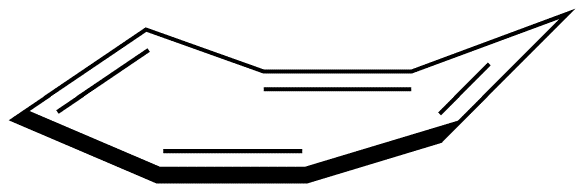A ring whose $\pi$ system contains 4n + 2 number of electrons, but is forced to deviate from planarity is also nonaromatic. This is why annulene (10) is nonaromatic.